# Average and Instantaneous Velocity & Speed

Average Velocity

The average velocity is the ratio of the total displacement and total time taken to complete the displacement. It should be noted that average velocity is independent of path as displacement is independent of path.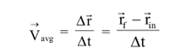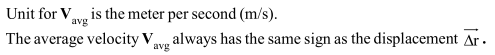Average Speed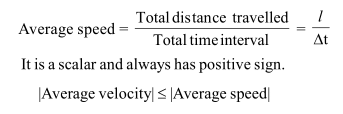Instantaneous Velocity

Instantaneous velocity is defined as the value approached by the average velocity when the time interval for measurement becomes closer and closer to zero.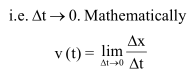Thus instantaneous velocity function is the derivative of the displacement with respect to time.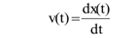Instantaneous Speed

It is measure of how fast a particle or a body is moving at a particular instant. It is the magnitude of instantaneous velocity. Thus particle moving with the instantaneous velocity of +5m/s and another moving with -5m/s will have same instantaneous speed of 5m/s.

The speedometer in a car measure the instantaneous speed not the instantaneous velocity, because it cannot determine the direction.

Post By : Divya SIngh 04 Feb, 2020 1424 views Physics